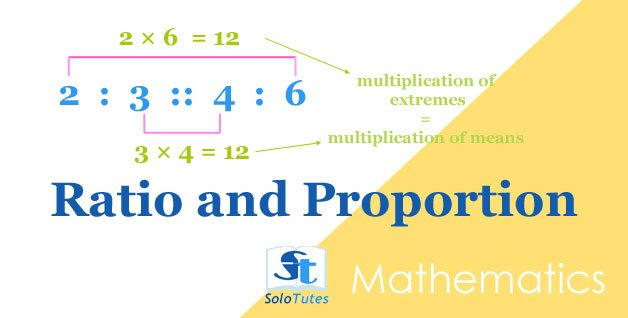SoloTutesClass 5th

Edit Class 5th ×Mathematics: Profit And Loss, Solved QuestionsMaNiSH manevandra 13-May-2020
The profit earned by selling an article for Rs 900 is double the loss incurred when the same article is sold for Rs 450. At what price should the article be sold to make a 25% profit?एक संख्या को जब 136 से भाग दिया जाता है, तो शेषफल 36 प्राप्त होता है। यदि इसी संख्या को 17 से भाग दिया जाए, तो शेषफल क्या होगा?SoloTutes Edu 11-Feb-2021Ratio and Proportion, Basic Tricks and Practice ProblemsSoloTutes 07-Apr-2021
ratio  can be written in the form of a :b ( where a and b are integers) is the simplified fractional relation ( a/b)  between two quantities which help us to find an easy interpretation about relative difference between two quantities. ration can be obtained by dividing one number to the another number of the same kind. proportion denotes the equality between two ratios.RatioSoloTutes 07-Apr-2021